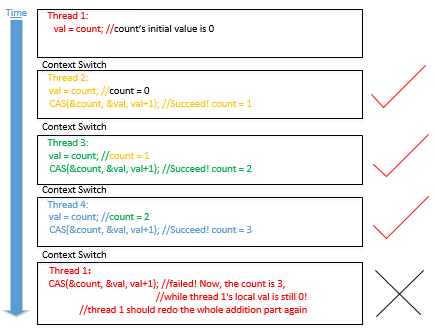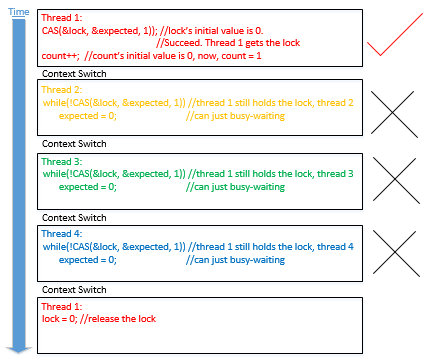# Lock-free vs spin-lock

So, given a piece of code, how do you know if it's lock-based or lock-free? Let's first see two examples. Both of them are multi-threads get access to a shared variable and do the addition on it. Here is the version by using lock-free programming.

``````1  #inlcude<stdio.h>
2  #include<stdatomic.h>
4
5  int count = 0;
7  {
8      int val;
9      for(int i=0; i<1000000; i++)
10     {
11         do{
12             val = count;
13         }while(!atomic_compare_exchange_weak(&count, &val, val+1));
14     }
16 }
17
18 int main()
19 {
21     for(int i=0; i<10; i++)
23     for(int i=0; i<10; i++)
25     printf("the value of count is %d\n", count);
26 }
``````

Here is the version of spin-lock for the same purpose.

``````1  #include<stdio.h>
2  #include<stdatomic.h>
4
5  int count = 0;
6  int lock = 0;
8  {
9      int expected = 0;
10     for(int i=0; i<1000000; i++)
11     {
12         while(!atomic_compare_exchange_weak(&lock, &expected, 1))//if the lock is 0(unlock), then set it to 1(lock)
13             expected = 0;//if the CAS fails, the expected will be set to 1, so we need to change it to 0 again.
14         count++;
15         lock = 0;
16     }
17 }
18
19 int main()
20 {
22     for(int i=0; i<10; i++)
24     for(int i=0; i<10; i++)
26     printf("the value of count is %d\n", count);
27 }
``````

Let's see the result(average result of running each program 10 times) and performance of the above two programs.

Lock-free:

``````the value of count is 10000000

Below is the running time:

real 0m2.687s
user 0m10.652s
sys  0m0.0004s
``````

Lock-based:

``````the value of count is 10000000

Below is the running time:

real 0m3.755s
user 0m14.820s
sys  0m0.012s
``````

From the above result, we could see that lock-free program is better than spin-lock program. Let's see the addition part of both programs.(For the lock-free program, it is the code from line 11 to 13, for the spin-lock program, it is the code from line 12 to 15) They're both loops, and very similarly-looking ones. Moreover, we can loop at both loops for a period of time. How come they're at the opposite sides of the locking/lock-free distinction?!

Let's first see an example.

lock-freelock-based